﻿ 利用扩展的(G'/G)展开法求(3 + 1)维YSFY势方程的精确解 Using the Extend (G'/G) Expansion Method to Obtain the Exact Solution of the (3 + 1)-Dimensional Potential YTSF Equation

Pure Mathematics
Vol. 09  No. 01 ( 2019 ), Article ID: 28694 , 8 pages
10.12677/PM.2019.91014

Using the Extend (G'/G) Expansion Method to Obtain the Exact Solution of the (3 + 1)-Dimensional Potential YTSF Equation

Houxu Mo, Yanfeng Guo, Pingli Zhu, Ganjie Liao

College of Science, Guangxi University of Science and Technology, Liuzhou Guangxi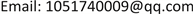Received: Jan. 4th, 2019; accepted: Jan. 22nd, 2019; published: Jan. 29th, 2019ABSTRACT

Using the extend (G'/G)-expansion method and the new auxiliary equations, the new exact solutions of (3 + 1)-dimensional potential Yu-Toda-Sasa-Fukuyama (YTSF) equation are obtained on the basis of the homogeneous balance method. And some forms of exact solutions of (3 + 1)-dimensional potential (YTSF) equation are given. Furthermore, the corresponding figures are given.

Keywords:(G'/G)-Expansion Methods, YTSF Equation, Exact Solution1. 前言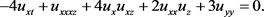(1)

${v}_{t}+\varphi \left(v\right){v}_{z}=0,\varphi \left(v\right)={\partial }_{x}^{2}+4v+2{v}_{x}{\partial }_{x}^{-1},$

$\left(-4{v}_{t}+\varphi \left(v\right){v}_{z}\right)+3{v}_{yy}=0,\varphi \left(v\right)={\partial }_{x}^{2}+4v+2{v}_{x}{\partial }_{x}^{-1},$

$AG{G}^{″}-BG{G}^{\prime }-C{\left({G}^{\prime }\right)}^{2}-E{G}^{2}=0,$ (2)

2. 扩展的 $\left({G}^{\prime }/G\right)$ 展开法的概述

1) 对于一般的非线性偏微分方程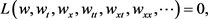(3)

$w\left(\zeta \right)=w\left(x,y,z,t\right),\zeta =ax+by+cz+{d}_{1}t,$ (4)

$a,b,c,{d}_{1}$ 为待定常数，将(4)代入(3)中，(3)就可化为

$Q\left({w}^{\prime },{w}^{″},{w}^{‴},{w}^{\left(4\right)},\cdots \right)=0.$ (5)

2) 设方程(5)的拟解为

$w\left(\xi \right)=\underset{g=-m}{\overset{m}{\sum }}{e}_{g}{\left(d+H\right)}^{g}+\underset{g=1}{\overset{m}{\sum }}{f}_{g}{\left(d+H\right)}^{g}.$ (6)

$AG{G}^{″}-BG{G}^{\prime }-C{\left({G}^{\prime }\right)}^{2}-E{G}^{2}=0,$ (7)

3) 将方程(6)和方程(7)代入方程(5)中，并将 $\left(d+H\right)$ 中相同的指数幂的系数合并，令各次幂的系数为零，得到一个关于 ${e}_{g},d,{f}_{g}\left(g=0,±1,±2,\cdots ,±m\right)$$a,b,c,{d}_{1}$$A,B,C,E$ 的代数方程组。

4) 利用maple软件求解代数方程组，确定待定常数之间的关系。

5) 通过文献  ，得到5组关于 $H\left(\xi \right)$ 的表达式

a) 当 $B\ne 0,\varphi =A-C$$\Omega ={B}^{2}+4E\varphi >0$ 时，

$H\left(\zeta \right)=\left(\frac{{G}^{\prime }}{G}\right)=\frac{B}{2\varphi }+\frac{\sqrt{\Omega }}{2\varphi }\frac{{C}_{1}\mathrm{sinh}\left(\frac{\sqrt{\Omega }}{2\varphi }\zeta \right)+{C}_{2}\mathrm{cosh}\left(\frac{\sqrt{\Omega }}{2\varphi }\zeta \right)}{{C}_{2}\mathrm{sinh}\left(\frac{\sqrt{\Omega }}{2\varphi }\zeta \right)+{C}_{1}\mathrm{cosh}\left(\frac{\sqrt{\Omega }}{2\varphi }\zeta \right)}$ (8)

b) 当 $B\ne 0,\varphi =A-C$$\Omega ={B}^{2}+4E\varphi <0$ 时，

$H\left(\zeta \right)=\left(\frac{{G}^{\prime }}{G}\right)=\frac{B}{2\varphi }+\frac{\sqrt{-\Omega }}{2\varphi }\frac{-{C}_{1}\mathrm{sin}\left(\frac{\sqrt{-\Omega }}{2\varphi }\zeta \right)+{C}_{2}\mathrm{cos}\left(\frac{\sqrt{-\Omega }}{2\varphi }\zeta \right)}{{C}_{2}\mathrm{sin}\left(\frac{\sqrt{-\Omega }}{2\varphi }\zeta \right)+{C}_{1}\mathrm{cos}\left(\frac{\sqrt{-\Omega }}{2\varphi }\zeta \right)}$ (9)

c) 当 $B\ne 0,\varphi =A-C$$\Omega ={B}^{2}+4E\varphi =0$ 时，

$H\left(\zeta \right)=\left(\frac{{G}^{\prime }}{G}\right)=\frac{B}{2\varphi }+\frac{{C}_{2}}{{C}_{1}+{C}_{2}\zeta }$ (10)

d) 当 $B=0,\varphi =A-C$$\Delta =\varphi E>0$ 时，

$H\left(\zeta \right)=\left(\frac{{G}^{\prime }}{G}\right)=\frac{\sqrt{\Delta }}{\varphi }\frac{{C}_{1}\mathrm{sinh}\left(\frac{\sqrt{\Delta }}{\varphi }\zeta \right)+{C}_{2}\mathrm{cosh}\left(\frac{\sqrt{\Delta }}{\varphi }\zeta \right)}{{C}_{2}\mathrm{sinh}\left(\frac{\sqrt{\Delta }}{\varphi }\zeta \right)+{C}_{1}\mathrm{cosh}\left(\frac{\sqrt{\Delta }}{\varphi }\zeta \right)}$ (11)

e) 当 $B=0,\varphi =A-C$$\Delta =\varphi E<0$ 时，

$H\left(\zeta \right)=\left(\frac{{G}^{\prime }}{G}\right)=\frac{\sqrt{\Delta }}{\varphi }\frac{-{C}_{1}\mathrm{sinh}\left(\frac{\sqrt{-\Delta }}{\varphi }\zeta \right)+{C}_{2}\mathrm{cosh}\left(\frac{\sqrt{-\Delta }}{\varphi }\zeta \right)}{{C}_{2}\mathrm{sinh}\left(\frac{\sqrt{-\Delta }}{\varphi }\zeta \right)+{C}_{1}\mathrm{cosh}\left(\frac{\sqrt{-\Delta }}{\varphi }\zeta \right)}$ (12)

3. Yu-Toda-Sasa-Fukuyama势方程的新精确解

$-4a{d}_{1}{w}^{″}+{a}^{3}c{w}^{\left(4\right)}+6{a}^{2}c{w}^{\prime }{w}^{″}+3{b}^{2}=0.$ (13)

$\left(-4a{d}_{1}+3{b}^{2}\right){w}^{\prime }+{a}^{3}c{w}^{‴}+3{a}^{2}c{\left({w}^{\prime }\right)}^{2}+k=0.$ (14)

$W\left(\zeta \right)={e}_{0}+\left({e}_{-1}+{f}_{1}\right){\left(d+H\right)}^{-1}+{e}_{1}\left(d+H\right)$ (15)

$a=a,b=b,c=c,d=d,k=0,{d}_{1}=\frac{1}{4}\frac{{a}^{3}c\Omega +3{A}^{2}{b}^{2}}{a{A}^{2}},{e}_{-1}=-\frac{2a{d}^{2}\phi +{f}_{1}A-2aE}{A},{e}_{1}=0,{f}_{1}={f}_{1}.$

$a=a,b=b,c=c,d=-\frac{1}{2}\frac{B}{\varphi },k=0,{d}_{1}=\frac{1}{4}\frac{4{a}^{3}c\Omega +3{A}^{2}{b}^{2}}{a{A}^{2}},{e}_{-1}=-\frac{1}{2}\frac{2A{f}_{1}\phi -a\Omega }{A\phi },{e}_{1}=\frac{2a\phi }{A},{f}_{1}={f}_{1}.$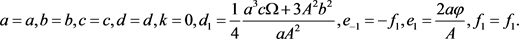${w}_{11}\left(\zeta \right)={e}_{0}+\left({e}_{-1}+{f}_{1}\right){\left(d+\frac{B}{2\phi }+\frac{\sqrt{\Omega }}{2\phi }\frac{{C}_{1}\mathrm{sinh}\left(\frac{\sqrt{\Omega }}{2\phi }\zeta \right)+{C}_{2}\mathrm{cosh}\left(\frac{\sqrt{\Omega }}{2\phi }\zeta \right)}{{C}_{2}\mathrm{sinh}\left(\frac{\sqrt{\Omega }}{2\phi }\zeta \right)+{C}_{1}\mathrm{cosh}\left(\frac{\sqrt{\Omega }}{2\phi }\zeta \right)}\right)}^{-1},$

${w}_{12}\left(\zeta \right)={e}_{0}+\left({e}_{-1}+{f}_{1}\right){\left(d+\frac{B}{2\phi }+\frac{\sqrt{-\Omega }}{2\phi }\frac{-{C}_{1}\mathrm{sin}\left(\frac{\sqrt{-\Omega }}{2\phi }\zeta \right)+{C}_{2}\mathrm{cos}\left(\frac{\sqrt{-\Omega }}{2\phi }\zeta \right)}{{C}_{2}\mathrm{sin}\left(\frac{\sqrt{-\Omega }}{2\phi }\zeta \right)+{C}_{1}\mathrm{cos}\left(\frac{\sqrt{-\Omega }}{2\phi }\zeta \right)}\right)}^{-1},$

${w}_{13}\left(\zeta \right)={e}_{0}+\left({e}_{-1}+{f}_{1}\right){\left(d+\frac{B}{2\phi }+\frac{{C}_{2}}{{C}_{1}+{C}_{2}\zeta }\right)}^{-1},$

${w}_{14}\left(\zeta \right)={e}_{0}+\left({e}_{-1}+{f}_{1}\right){\left(d+\frac{\sqrt{\Delta }}{M}\frac{{C}_{1}\mathrm{sinh}\left(\frac{\sqrt{\Delta }}{\phi }\zeta \right)+{C}_{2}\mathrm{cosh}\left(\frac{\sqrt{\Delta }}{\phi }\zeta \right)}{{C}_{2}\mathrm{sinh}\left(\frac{\sqrt{\Delta }}{\phi }\zeta \right)+{C}_{1}\mathrm{cosh}\left(\frac{\sqrt{\Delta }}{\phi }\zeta \right)}\right)}^{-1},$

${w}_{15}\left(\zeta \right)={e}_{0}+\left({e}_{-1}+{f}_{1}\right){\left(d+\frac{\sqrt{-\Delta }}{\phi }\frac{-{C}_{1}\mathrm{sin}\left(\frac{\sqrt{-\Delta }}{\phi }\zeta \right)+{C}_{2}\mathrm{cos}\left(\frac{\sqrt{-\Delta }}{\phi }\zeta \right)}{{C}_{2}\mathrm{sin}\left(\frac{\sqrt{-\Delta }}{\phi }\zeta \right)+{C}_{1}\mathrm{cos}\left(\frac{\sqrt{-\Delta }}{\phi }\zeta \right)}\right)}^{-1}.$

$\begin{array}{c}{w}_{21}\left(\zeta \right)={e}_{0}+\left({e}_{-1}+{f}_{1}\right){\left(d+\frac{B}{2\phi }+\frac{\sqrt{\Omega }}{2\phi }\frac{{C}_{1}\mathrm{sinh}\left(\frac{\sqrt{\Omega }}{2\phi }\zeta \right)+{C}_{2}\mathrm{cosh}\left(\frac{\sqrt{\Omega }}{2\phi }\zeta \right)}{{C}_{2}\mathrm{sinh}\left(\frac{\sqrt{\Omega }}{2\phi }\zeta \right)+{C}_{1}\mathrm{cosh}\left(\frac{\sqrt{\Omega }}{2\phi }\zeta \right)}\right)}^{-1}\\ +{e}_{1}\left(d+\frac{B}{2\phi }+\frac{\sqrt{\Omega }}{2\phi }\frac{{C}_{1}\mathrm{sinh}\left(\frac{\sqrt{\Omega }}{2\phi }\zeta \right)+{C}_{2}\mathrm{cosh}\left(\frac{\sqrt{\Omega }}{2\phi }\zeta \right)}{{C}_{2}\mathrm{sinh}\left(\frac{\sqrt{\Omega }}{2\phi }\zeta \right)+{C}_{1}\mathrm{cosh}\left(\frac{\sqrt{\Omega }}{2\phi }\zeta \right)}\right),\end{array}$

$\begin{array}{c}{w}_{22}\left(\zeta \right)={e}_{0}+\left({e}_{-1}+{f}_{1}\right){\left(d+\frac{B}{2\phi }+\frac{\sqrt{-\Omega }}{2\phi }\frac{-{C}_{1}\mathrm{sin}\left(\frac{\sqrt{-\Omega }}{2\phi }\zeta \right)+{C}_{2}\mathrm{cos}\left(\frac{\sqrt{-\Omega }}{2\phi }\zeta \right)}{{C}_{2}\mathrm{sin}\left(\frac{\sqrt{-\Omega }}{2\phi }\zeta \right)+{C}_{1}\mathrm{cos}\left(\frac{\sqrt{-\Omega }}{2\phi }\zeta \right)}\right)}^{-1}\\ +{e}_{1}\left(d+\frac{B}{2\phi }+\frac{\sqrt{-\Omega }}{2\phi }\frac{-{C}_{1}\mathrm{sin}\left(\frac{\sqrt{-\Omega }}{2\phi }\zeta \right)+{C}_{2}\mathrm{cos}\left(\frac{\sqrt{-\Omega }}{2\phi }\zeta \right)}{{C}_{2}\mathrm{sin}\left(\frac{\sqrt{-\Omega }}{2\phi }\zeta \right)+{C}_{1}\mathrm{cos}\left(\frac{\sqrt{-\Omega }}{2\phi }\zeta \right)}\right),\end{array}$

${w}_{23}\left(\zeta \right)={e}_{0}+\left({e}_{-1}+{f}_{1}\right){\left(d+\frac{B}{2\phi }+\frac{{C}_{2}}{{C}_{1}+{C}_{2}\zeta }\right)}^{-1}+{e}_{1}\left(d+\frac{B}{2\phi }+\frac{{C}_{2}}{{C}_{1}+{C}_{2}\zeta }\right),$

$\begin{array}{c}{w}_{24}\left(\zeta \right)={e}_{0}+\left({e}_{-1}+{f}_{1}\right){\left(d+\frac{\sqrt{\Delta }}{M}\frac{{C}_{1}\mathrm{sinh}\left(\frac{\sqrt{\Delta }}{\phi }\zeta \right)+{C}_{2}\mathrm{cosh}\left(\frac{\sqrt{\Delta }}{\phi }\zeta \right)}{{C}_{2}\mathrm{sinh}\left(\frac{\sqrt{\Delta }}{\phi }\zeta \right)+{C}_{1}\mathrm{cosh}\left(\frac{\sqrt{\Delta }}{\phi }\zeta \right)}\right)}^{-1}\\ +{e}_{1}\left(d+\frac{\sqrt{\Delta }}{M}\frac{{C}_{1}\mathrm{sinh}\left(\frac{\sqrt{\Delta }}{\phi }\zeta \right)+{C}_{2}\mathrm{cosh}\left(\frac{\sqrt{\Delta }}{\phi }\zeta \right)}{{C}_{2}\mathrm{sinh}\left(\frac{\sqrt{\Delta }}{\phi }\zeta \right)+{C}_{1}\mathrm{cosh}\left(\frac{\sqrt{\Delta }}{\phi }\zeta \right)}\right),\end{array}$

$\begin{array}{c}{w}_{25}\left(\zeta \right)={e}_{0}+\left({e}_{-1}+{f}_{1}\right){\left(d+\frac{\sqrt{-\Delta }}{\phi }\frac{-{C}_{1}\mathrm{sin}\left(\frac{\sqrt{-\Delta }}{\phi }\zeta \right)+{C}_{2}\mathrm{cos}\left(\frac{\sqrt{-\Delta }}{\phi }\zeta \right)}{{C}_{2}\mathrm{sin}\left(\frac{\sqrt{-\Delta }}{\phi }\zeta \right)+{C}_{1}\mathrm{cos}\left(\frac{\sqrt{-\Delta }}{\phi }\zeta \right)}\right)}^{-1}\\ +{e}_{1}\left(d+\frac{\sqrt{-\Delta }}{\phi }\frac{-{C}_{1}\mathrm{sin}\left(\frac{\sqrt{-\Delta }}{\phi }\zeta \right)+{C}_{2}\mathrm{cos}\left(\frac{\sqrt{-\Delta }}{\phi }\zeta \right)}{{C}_{2}\mathrm{sin}\left(\frac{\sqrt{-\Delta }}{\phi }\zeta \right)+{C}_{1}\mathrm{cos}\left(\frac{\sqrt{-\Delta }}{\phi }\zeta \right)}\right).\end{array}$

${w}_{31}\left(\zeta \right)={e}_{0}+{e}_{1}\left(d+\frac{B}{2\phi }+\frac{\sqrt{\Omega }}{2\phi }\frac{{C}_{1}\mathrm{sinh}\left(\frac{\sqrt{\Omega }}{2\phi }\zeta \right)+{C}_{2}\mathrm{cosh}\left(\frac{\sqrt{\Omega }}{2\phi }\zeta \right)}{{C}_{2}\mathrm{sinh}\left(\frac{\sqrt{\Omega }}{2\phi }\zeta \right)+{C}_{1}\mathrm{cosh}\left(\frac{\sqrt{\Omega }}{2\phi }\zeta \right)}\right),$

${w}_{32}\left(\zeta \right)={e}_{0}+{e}_{1}\left(d+\frac{B}{2\phi }+\frac{\sqrt{-\Omega }}{2\phi }\frac{-{C}_{1}\mathrm{sin}\left(\frac{\sqrt{-\Omega }}{2\phi }\zeta \right)+{C}_{2}\mathrm{cos}\left(\frac{\sqrt{-\Omega }}{2\phi }\zeta \right)}{{C}_{2}\mathrm{sin}\left(\frac{\sqrt{-\Omega }}{2\phi }\zeta \right)+{C}_{1}\mathrm{cos}\left(\frac{\sqrt{-\Omega }}{2\phi }\zeta \right)}\right),$

${w}_{33}\left(\zeta \right)={e}_{0}+{e}_{1}\left(d+\frac{B}{2\phi }+\frac{{C}_{2}}{{C}_{1}+{C}_{2}\zeta }\right),$

${w}_{34}\left(\zeta \right)={e}_{0}+{e}_{1}\left(d+\frac{\sqrt{\Delta }}{M}\frac{{C}_{1}\mathrm{sinh}\left(\frac{\sqrt{\Delta }}{\phi }\zeta \right)+{C}_{2}\mathrm{cosh}\left(\frac{\sqrt{\Delta }}{\phi }\zeta \right)}{{C}_{2}\mathrm{sinh}\left(\frac{\sqrt{\Delta }}{\phi }\zeta \right)+{C}_{1}\mathrm{cosh}\left(\frac{\sqrt{\Delta }}{\phi }\zeta \right)}\right),$

${w}_{35}\left(\zeta \right)={e}_{0}+{e}_{1}\left(d+\frac{\sqrt{-\Delta }}{\phi }\frac{-{C}_{1}\mathrm{sin}\left(\frac{\sqrt{-\Delta }}{\phi }\zeta \right)+{C}_{2}\mathrm{cos}\left(\frac{\sqrt{-\Delta }}{\phi }\zeta \right)}{{C}_{2}\mathrm{sin}\left(\frac{\sqrt{-\Delta }}{\phi }\zeta \right)+{C}_{1}\mathrm{cos}\left(\frac{\sqrt{-\Delta }}{\phi }\zeta \right)}\right).$

$r={\alpha }_{1}x+{\alpha }_{2}y+{\alpha }_{3}z$ ( ${\alpha }_{1},{\alpha }_{2},{\alpha }_{3}$ 为不为零的常数)，利用maple软件画出部分解的图像，如下：(图1~图3)$\begin{array}{l}{w}_{12}\left(\zeta \right):a=C={C}_{1}=d=1,b=4,c=16,{d}_{1}=8,{C}_{2}=A=B=2,E={f}_{1}=-2,\\ {e}_{-1}=-3,{e}_{1}=0,{e}_{0}=-1.\end{array}$

Figure 1. Triangle function solution $\begin{array}{l}{w}_{12}\left(\zeta \right)\end{array}$ schematic diagram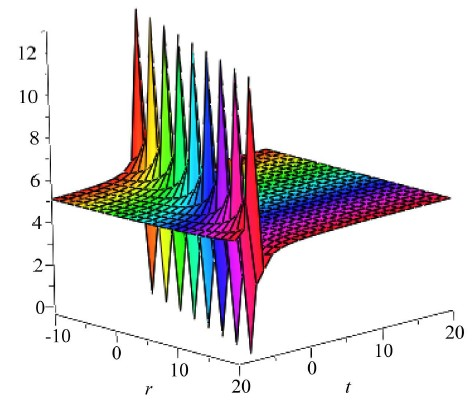${w}_{33}\left(\zeta \right):a=A=E={C}_{1}={e}_{0}=1,b=c=B={C}_{2}=2,d=0,{d}_{1}=3,{e}_{1}=-4.$

Figure 2. Rational partition solution ${w}_{33}\left(\zeta \right)$ schematic diagram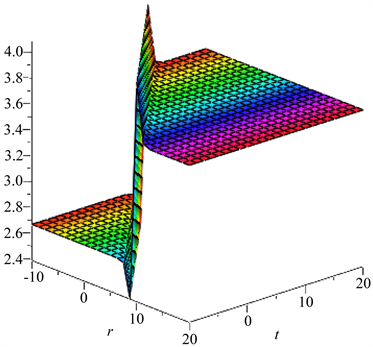$\begin{array}{l}{w}_{24}\left(\zeta \right):a=b=c={f}_{1}={C}_{1}={d}_{1}={e}_{0}=1,d=2,A=4,{C}_{2}=C=2,B=0,\\ E=\frac{1}{2},{e}_{1}=1,{e}_{-1}=-\frac{3}{4}.\end{array}$

Figure 3. Hyperbolic function solution ${w}_{24}\left(\zeta \right)$ schematic diagram

4. 结论

Using the Extend (G'/G) Expansion Method to Obtain the Exact Solution of the (3 + 1)-Dimensional Potential YTSF Equation[J]. 理论数学, 2019, 09(01): 111-118. https://doi.org/10.12677/PM.2019.91014

1. 1. 王元明. 数学物理方程与特殊函数(第三版) [M]. 北京: 高等教育出版社, 2004.

2. 2. 傅海明, 戴正德. (2+1)维广义KdV方程的周期孤波解[J]. 平顶山学院学报, 2013, 28(5): 35-38.

3. 3. 刘建国, 曾志芳. 变系数Sine-Gordon 方程的Bäcklund变换和新的精确解[J]. 系统科学与数学, 2014, 34(6): 763-768.

4. 4. 傅海明, 戴正德. (2+1)维Nizhnik-Novikov方程的周期孤波解[J]. 江苏师范学报(自然科学版), 2016, 34(2): 33-36.

5. 5. Kongl, Q. and Dai, C.Q. (2015) Some Discussions about Variable Separation of Nonlinear Models Using Riccati Equation Expansion Method. Nonlinear Dynamics, 81, 1553-1561.
https://doi.org/10.1007/s11071-015-2089-y

6. 6. Yu, S.J., Toda, K., Sasa, N. and Fukuyama, T. (1998) N-Solitions to the Bo-goyavlenskii-Schiff Equation and Aquest for the Solition in (3+1)-Dimensions. Journal of Physics A, 31, 3337.
https://doi.org/10.1088/0305-4470/31/14/018

7. 7. Schff, J. and Painleve, T. (1992) Their Asymptotics and Physical Applica-tions. Plenum, New York.

8. 8. Vladimirov, V.A. and Maczka, C. (2007) Exact Solutions of Generalized Burgers Equation, De-scribing Travelling Fronts and Their Interaction. Reports on Mathematical Physics, 60, 317-328.
https://doi.org/10.1016/S0034-4877(07)80142-X

9. 9. Darvishi, M.T., Najafi, M., Kavitha, L. and Venkatesh, M. (2012) Stair and Step Solition Solutions of the Integrable (2+1) and (3+1)-Dimensional Boiti-Leon-Manna-Pempinelli Equations. Communications in Theoretical Physics, 58, 785-794.
https://doi.org/10.1088/0253-6102/58/6/01

10. 10. Tan, W. and Dai, Z.D. (2016) Dynamics of Kinky Wave for (3+1)- Dimensional Potential Yu-Toda-Sasa-Fukuyama. Nonlinear Dynamics, 85, 817-823.
https://doi.org/10.1007/s11071-016-2725-1

11. 11. Yun, Z. (2003) New Families of Nontravelling Wave Solutions to a New (3+1)-Dimensional Potential-YTSF Equation. Physics Letters A, 318, 78-83.
https://doi.org/10.1016/j.physleta.2003.08.073

12. 12. Zhang, T., Xuan, H.N., Zhang, D.F. and Wang, C.J. (2007) Nontraveling Wave Solutions to a (3+1) Dimensional Potential YTSF Equation and a Simplified Model for Reacting Mixtures. Chaos, Solitions Fractals, 34, 1006-1013.
https://doi.org/10.1016/j.chaos.2006.04.005

13. 13. Hasibun, N. and Farah, A. (2014) New Generalized and Improved (G'/G)-Expansion Method for Nonlinear Evolution Equations in Mathematical Physic. Journal of the Egyptian Mathematical Society, 22, 390-395.
https://doi.org/10.1016/j.joems.2013.11.008